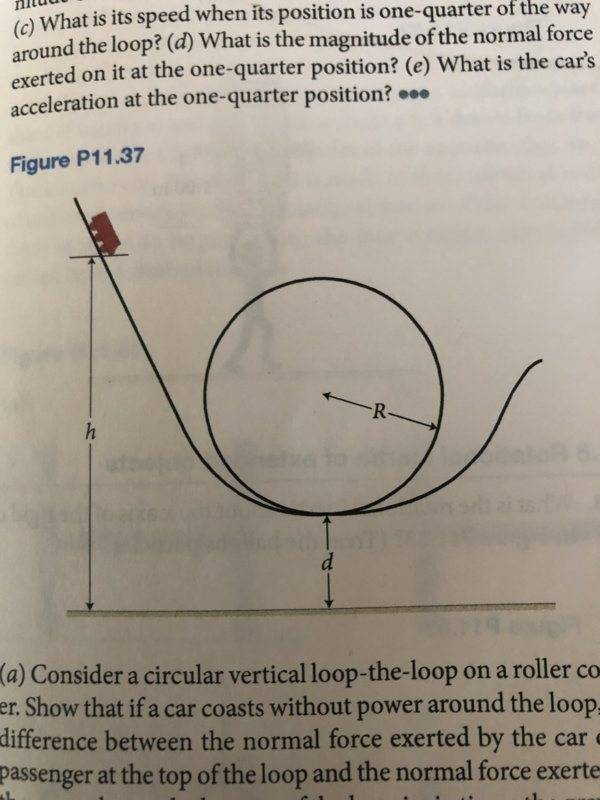# Roller Coaster Loop Problem

• Jaccobtw
In summary, the conversation discusses the normal force and gravity acting on a car on a curved track, with the discovery that the normal force is greater than the force due to gravity. The conversation also touches on the car's acceleration at the one-quarter position and potential errors in the given answer not including the gravitational component.

#### Jaccobtw

Homework Statement
A roller-coaster car initially at position a position on the track a height h above the ground begins a downward run on a long, steeply sloping track and then goes into a circular loop of radius R whose bottom is a distance d above the ground. Ignore friction. What is the magnitude of the normal force exerted on the car at the bottom of the loop?
Relevant Equations
F = ma
K = (1/2)mv^2
a = v^2/rSo, we know that at the bottom of the loop, the car will have a normal force pointing upward and gravity pointing down. However, I have discovered that the normal force is apparently greater than the force due to gravity.

Basically

N = F(g) + ?

What is this other force?

The cart is on a curved track so it is not in equilibrium.

oh wait, then how am I supposed to express the magnitude of the normal force?

Orodruin said:
The cart is on a curved track so it is not in equilibrium.

By the way, congrats on your prestige.

Ok I just got mg(1+(2h-2d)/R) for the Normal force.

Apparently, this force -----> mv^2/R is a completely separate force from mg

Here's what I did:

I added mg to mv^2/R, but v is expressed as sqrt(2g(h-d)) from the previous question not shown.

Jaccobtw said:
Ok I just got mg(1+(2h-2d)/R) for the Normal force.

Apparently, this force -----> mv^2/R is a completely separate force from mg

Here's what I did:

I added mg to mv^2/R, but v is expressed as sqrt(2g(h-d)) from the previous question not shown.
Looks right.

•Jaccobtw
The next question asks: What is the car's acceleration at the one-quarter position?

And I got this : (2g(h-R-d))/(R)

And the back of the book says this is correct, however, I just realized this answer does not include acceleration due to gravity, only the normal force. Isn't the net acceleration at this point in the circle down and to the left?

Something like (2g(h-R-d))/(R * cos(theta)) or g/sin(theta)

If not, what is wrong with these two answers?

Jaccobtw said:
The next question asks: What is the car's acceleration at the one-quarter position?

And I got this : (2g(h-R-d))/(R)

And the back of the book says this is correct, however, I just realized this answer does not include acceleration due to gravity, only the normal force. Isn't the net acceleration at this point in the circle down and to the left?

Something like (2g(h-R-d))/(R * cos(theta)) or g/sin(theta)

If not, what is wrong with these two answers?
I agree that the given answer is incorrect. It ought to include a gravitational component. But to do that, use Pythagoras.

•Jaccobtw and hmmm27